# 具有初值间断的Burgers方程奇摄动解*

(1. 杭州电子科技大学 理学院, 杭州 310018;2. 杭州电子科技大学 机械工程学院, 杭州 310018)

## 1 模 型 建 立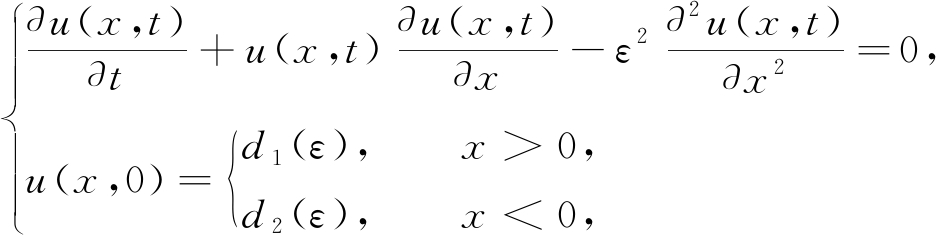(1)

(H1) (x,t)∈(-∞,+∞)×[0,T],其中T是有限值;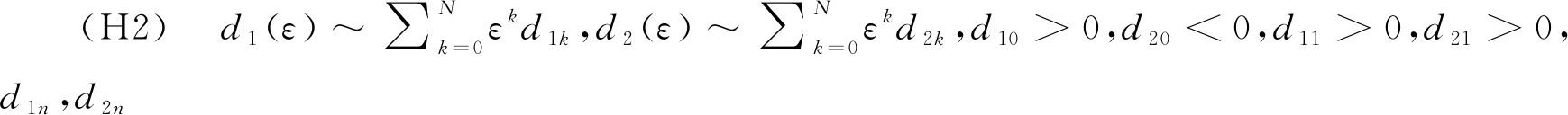表示给定的初始值且均为常数

## 2 形 式 展 开

### 2.1 外部解形式渐近展开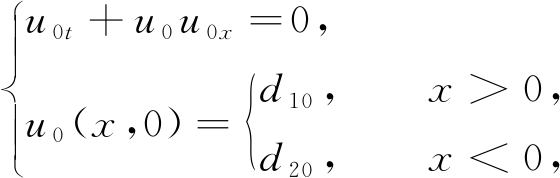(2)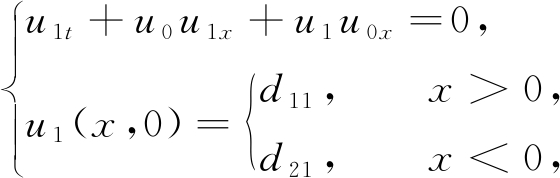(3)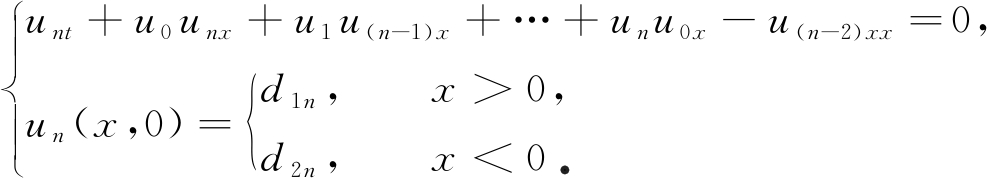(4)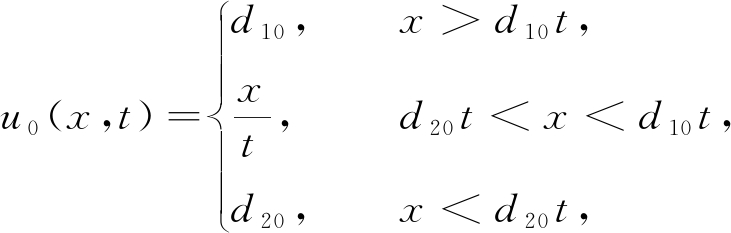(5)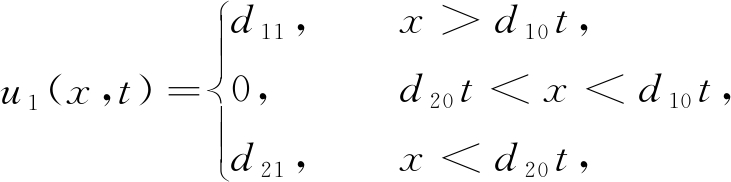(6)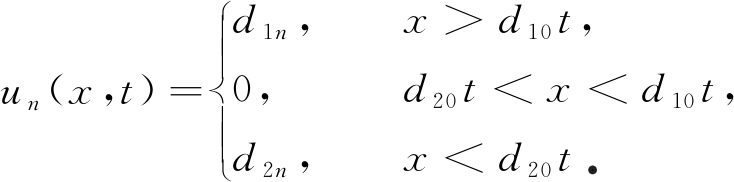(7)

### 2.2 内部解形式渐近展开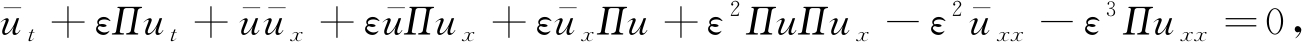(8)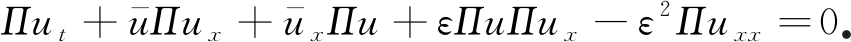(9)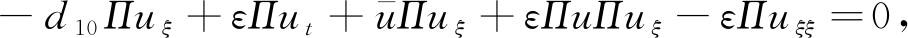(10)

ε的幂指数形式展开，可得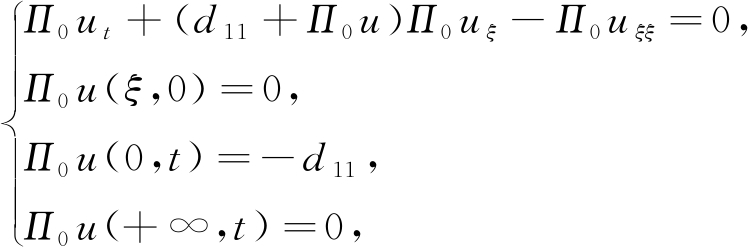(11)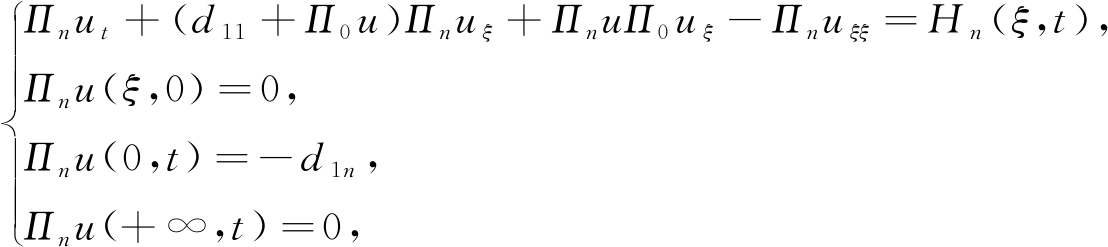(12)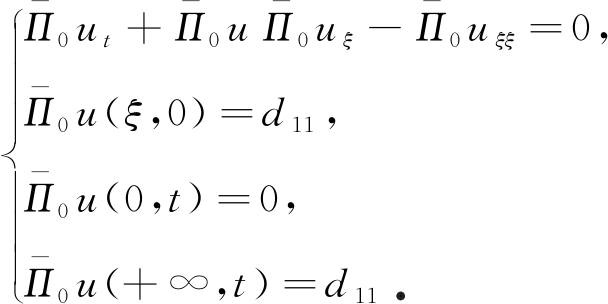(13)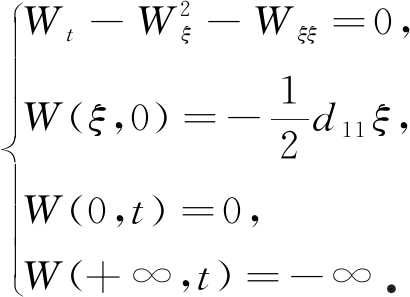(14)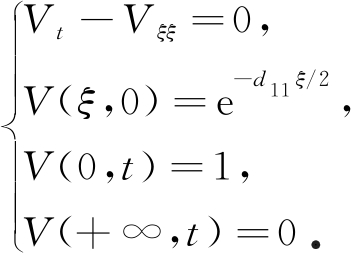(15)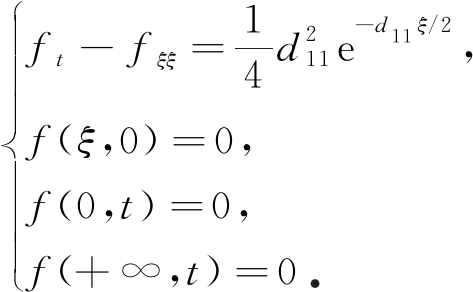(16)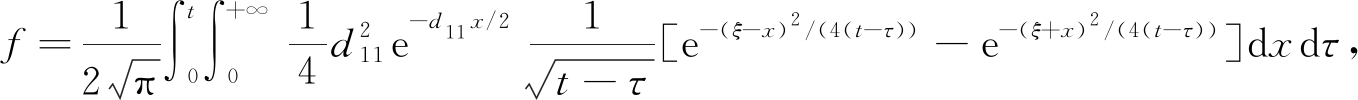(17)

V=e-d11ξ/2+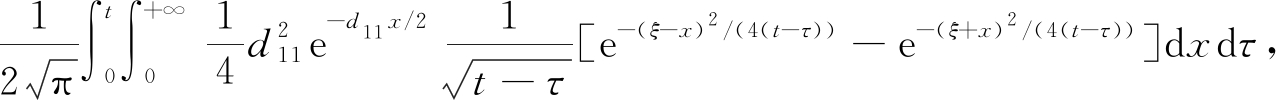(18)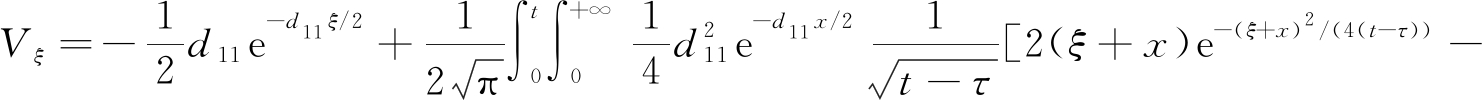2(ξ-x)e-(ξ-x)2/(4(t-τ))]dxdτ,

(19)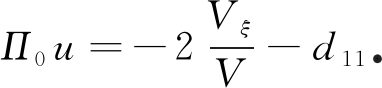(20)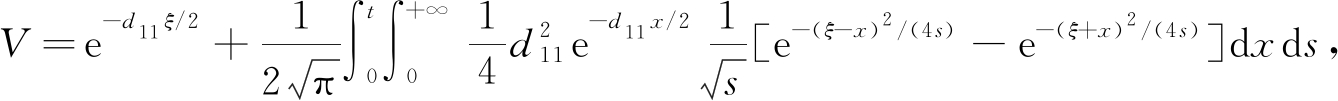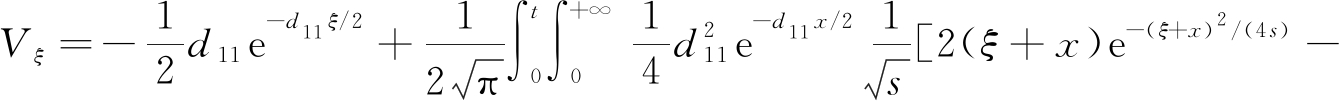2(ξ-x)e-(ξ-x)2/(4s)]dxds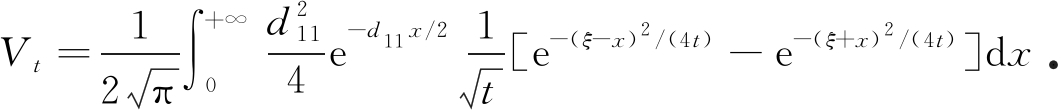e-(ξ-x)2/(4s)-e-(ξ+x)2/(4s)=e-(ξ+x)2/(4s)(eξx/s-1)≥0，

V>0，Vt>0则VVt>0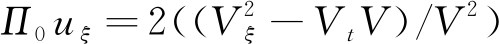可得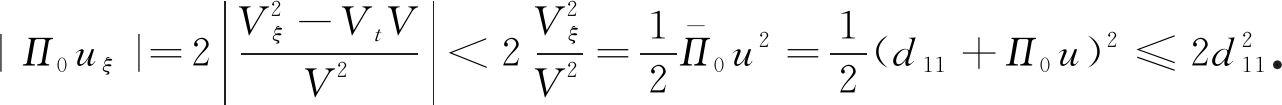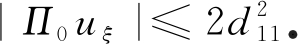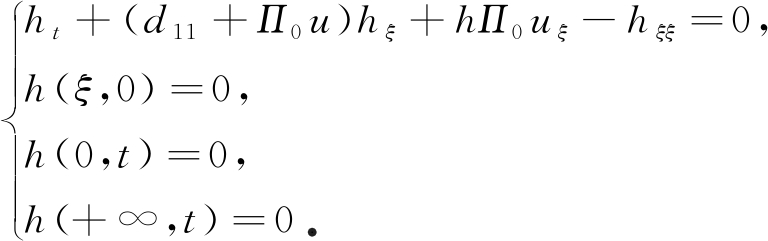(21)

h=eλtZ，则ht=eλtZt+λeλtZhξ=eλtZξ,hξξ=eλtZξξ,式(21)可变为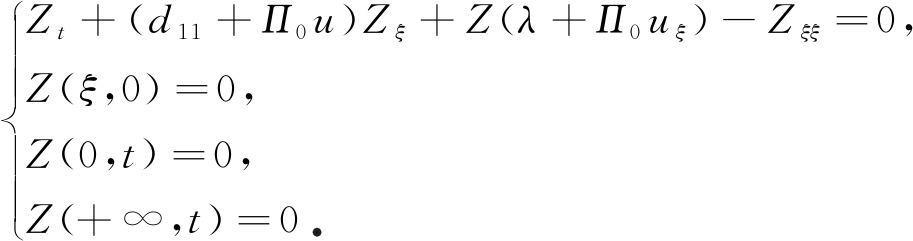(22)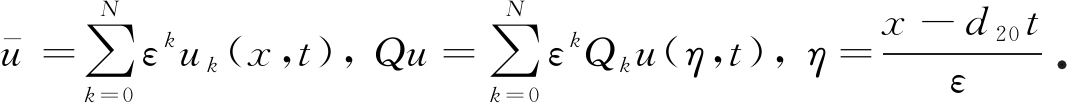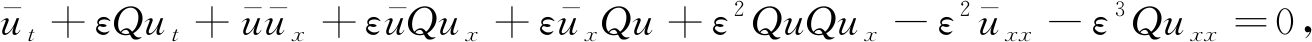(23)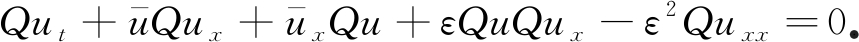(24)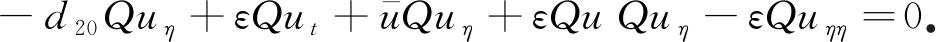(25)

ε的幂指数形式展开，可得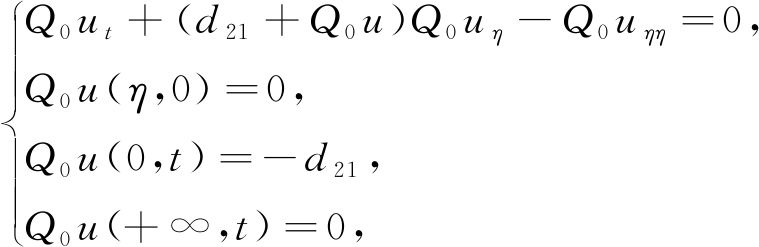(26)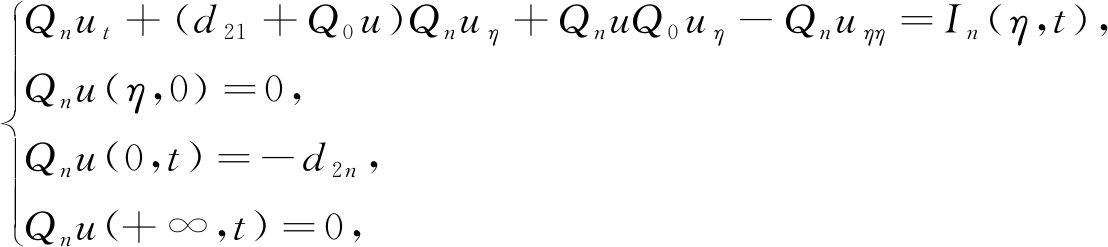(27)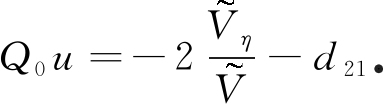(28)

## 3 余 项 估 计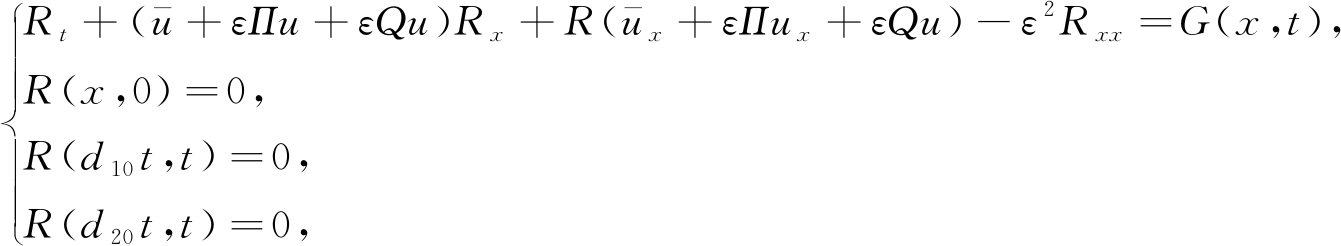(29)

R=eβtJ，则Rt=eβtJt+βeβtJRx=eβtJx,Rxx=eβtJxx，其中取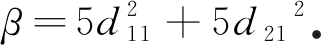式(29)可变为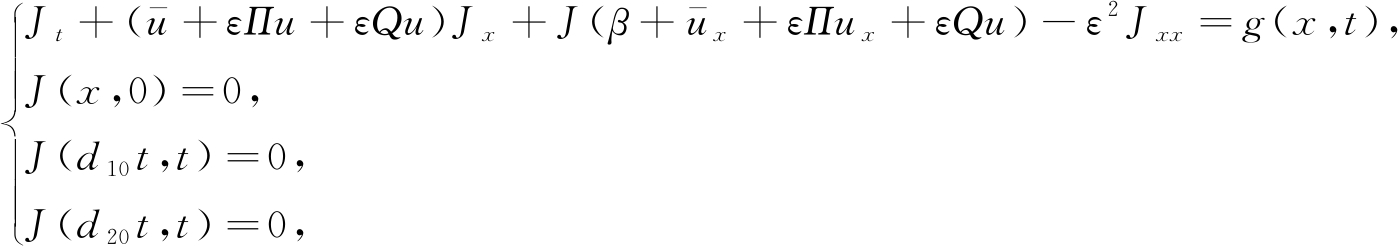(30)

1) 在区域Ω1={(x,t)∈R×[0,T]|xd10t}上，有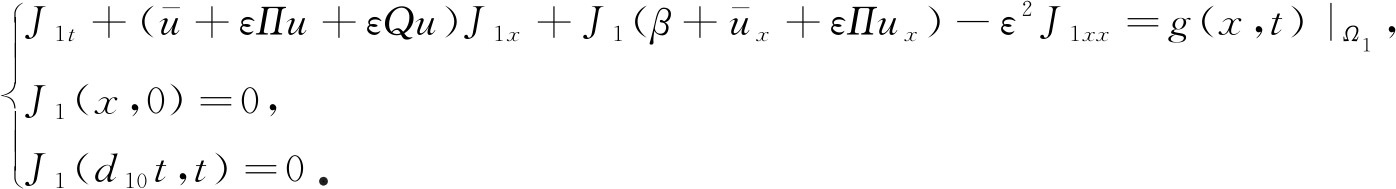(31)

t0=0，x0=d10t时，由定解条件可得J1(x,0)=0；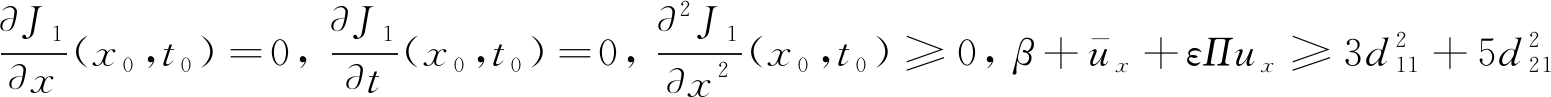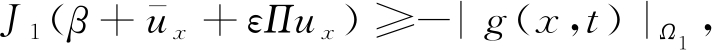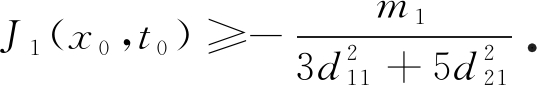2) 在区域Ω2={(x,t)∈R×[0,T]|d20txd10t}上，有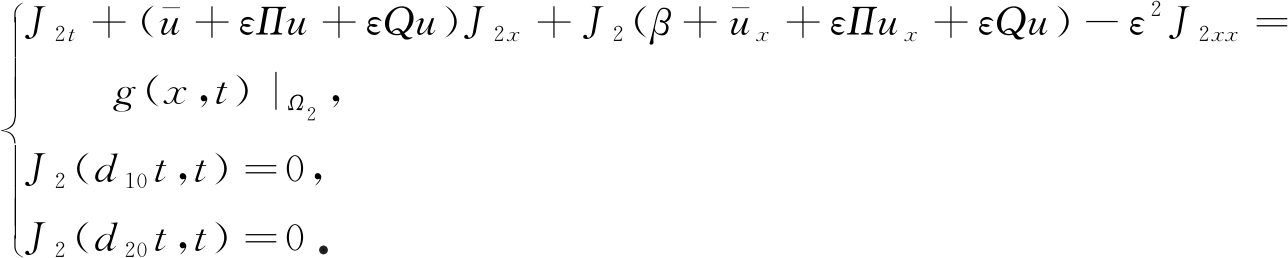(32)

x0=d10tx0=d20t时，由定解条件可得J2(x,0)=0;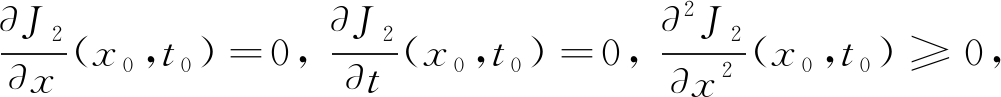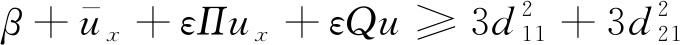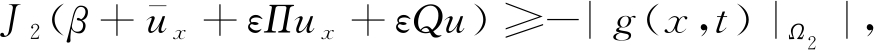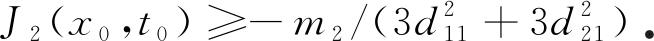3) 在区域Ω3={(x,t)∈R×[0,T]|xd20t}上，有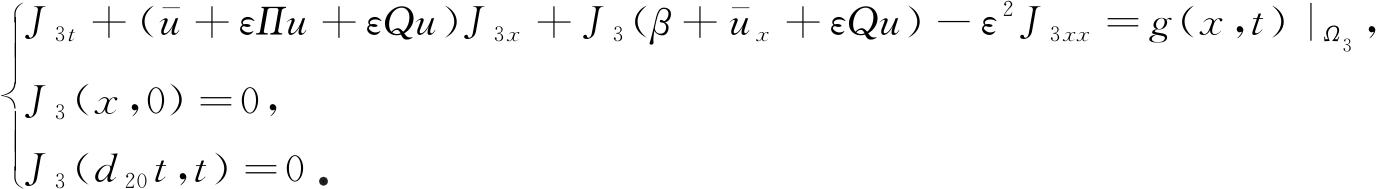(33)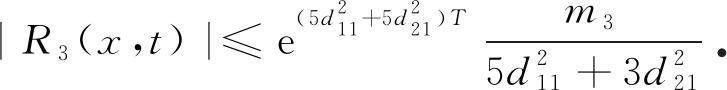|R(x,t)|≤M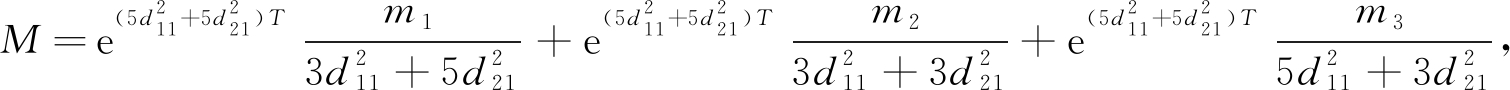R有界，即形式渐近解一致有效

## 4 结 束 语

 SEN A, RAJA SEKHAR T. Delta shock wave as self-similar viscosity limit for a strictly hyperbolic system of conservation laws[J].Journal of Mathematical Physics, 2019,60(5): 051510.

 GALAKTIONOV V A. On self-similar collapse of discontinuous data for thin film equations with doubly degenerate mobility[R/OL]. 2009. [2019-09-12]. http://citeseerx.ist.psu.edu/viewdoc/download?doi=10.1.1.243.8638& rep=rep1& type=pdf.

 瞿霞. 流体力学中Euler方程组的Riemann问题[D]. 硕士学位论文. 上海: 上海师范大学, 2019.(QU Xia. Riemann problem of Euler equations in fluid mechanics[D]. Master Thesis. Shanghai: Shanghai Normal University, 2019.(in Chinese))

 SHEN C. The Riemann problem for the pressureless Euler system with the Coulomb-like friction term[J].IMA Journal of Applied Mathematics, 2015,81(1): 76-99.

 WANG L. The Riemann problem with delta data for zero-pressure gas dynamics[J].Chinese Annals of Mathematics(Series B), 2016,37(3): 441-450.

 ZHANG Y H, PAN R H, TAN Z. Zero dissipation limit to a Riemann solution consisting of two shock waves for the 1D compressible isentropic Navier-Stokes equations[J].Science China:Mathematics, 2013,56(11): 2205-2232.

 HUANG F, WANG Y, YANG T. Vanishing viscosity limit of the compressible Navier-Stokes equations for solutions to a Riemann problem[J].Archive for Rational Mechanics and Analysis, 2012,203(2): 379-413.

 CHEN Z, XIONG L, MENG Y J. Convergence to the superposition of rarefaction waves and contact discontinuity for the 1-D compressible Navier-Stokes-Korteweg system[J].Journal of Mathematical Analysis and Applications, 2014,412(2): 646-663.

 CHEN Z Z, CHAI X J, WANG W J. Convergence rate of solutions to strong contact discontinuity for the one-dimensional compressible radiation hydrodynamics model[J].Acta Mathematica Scientia, 2016,36(1): 265-282.

 YOSHIA Z. Singular perturbation and scale hierarchy in plasma flows[C]//Autumn College on Plasma Physics:Long-Lived Structures and Self Organization in Plasmas. Trieste, Italy, 2003.

 FERDOUSI M, YASMIN S, ASHRAF S, et al. Cylindrical and spherical ion-acoustic shock waves in nonextensive electron-positron-ion plasma[J].IEEE Transactions on Plasma Science, 2015,43(2): 643-649.

 YANG X J, GAO F, SRIVASTAVA H M. Exact travelling wave solutions for the local fractional two-dimensional Burgers-type equations[J].Computers&Mathematics With Applications, 2017,73(2): 203-210.

 SEADAWY A R. Ion acoustic solitary wave solutions of two-dimensional nonlinear Kadomtsev-Petviashvili-Burgers equation in quantum plasma[J].Mathematical Methods in the Applied Sciences, 2017,40(5): 1598-1607.

 FANG B, TANG P, WANG Y G. The Riemann problem of the Burgers equation with a discontinuous source term[J].Journal of Mathematical Analysis and Applications, 2012,395(1): 307-335.

 拉奥 C S, 亚达夫 M K. 非齐次Burgers方程解的渐近性行为[J]. 应用数学和力学, 2010,31(9): 1133-1139. (RAO C S, YADAV M K. Asymptotic behavior of solutions to nonhomogeneous Burgers equation[J].Applied Mathematics and Mechanics, 2010,31(9): 1133-1139.(in Chinese))

 伍卓群, 尹景学, 王春明. 椭圆与抛物型方程引论[M]. 北京: 科学出版社, 2003.(WU Zhuoqun, YIN Jingxue, WANG Chunming.Introduction to Elliptic and Parabolic Equations[M]. Beijing: Science Press, 2003.(in Chinese))

# Singularly Perturbed Solutions of Burgers Equations With Initial Value Discontinuities

BAO Liping1, HU Yubo1, WU Liqun2

(1.School of Sciences,Hangzhou Dianzi University,Hangzhou310018,P.R.China;2.School of Mechanical Engineering,Hangzhou Dianzi University,Hangzhou310018,P.R.China)

Abstract:The wave model generated for laser plasma was discussed, which can be expressed as the Riemann problem of Burgers equations with initial value discontinuity. The singularly perturbed asymptotic solution of the Burgers equations with discontinuous initial values was obtained with the singularly perturbed expansion method. The solution was divided into 2 parts: an outer solution and an inner layer correction term. Since the initial condition is constant, the wave will generate the characteristic boundary in the process of propagation, and the correction term will make the parabolic characteristic boundary. The external solution was corrected at the internal layer along the characteristic lines. The existence and uniqueness of the asymptotic solution was proved through the Hopf-Cole transform, Fourier transform and the extremum principle. Then the asymptotic expansion is obtained with the uniform validity proved.

Key words:Burgers equation; discontinuous initial value; characteristic line; singular perturbation; uniform validity estimation

DOI:10.21656/1000-0887.400270

ⓒ 应用数学和力学编委会，ISSN 1000-0887

http://www.applmathmech.cn

*收稿日期： 2019-09-12；修订日期：2019-11-04

Foundation item:The National Natural Science Foundation of China(51775154)